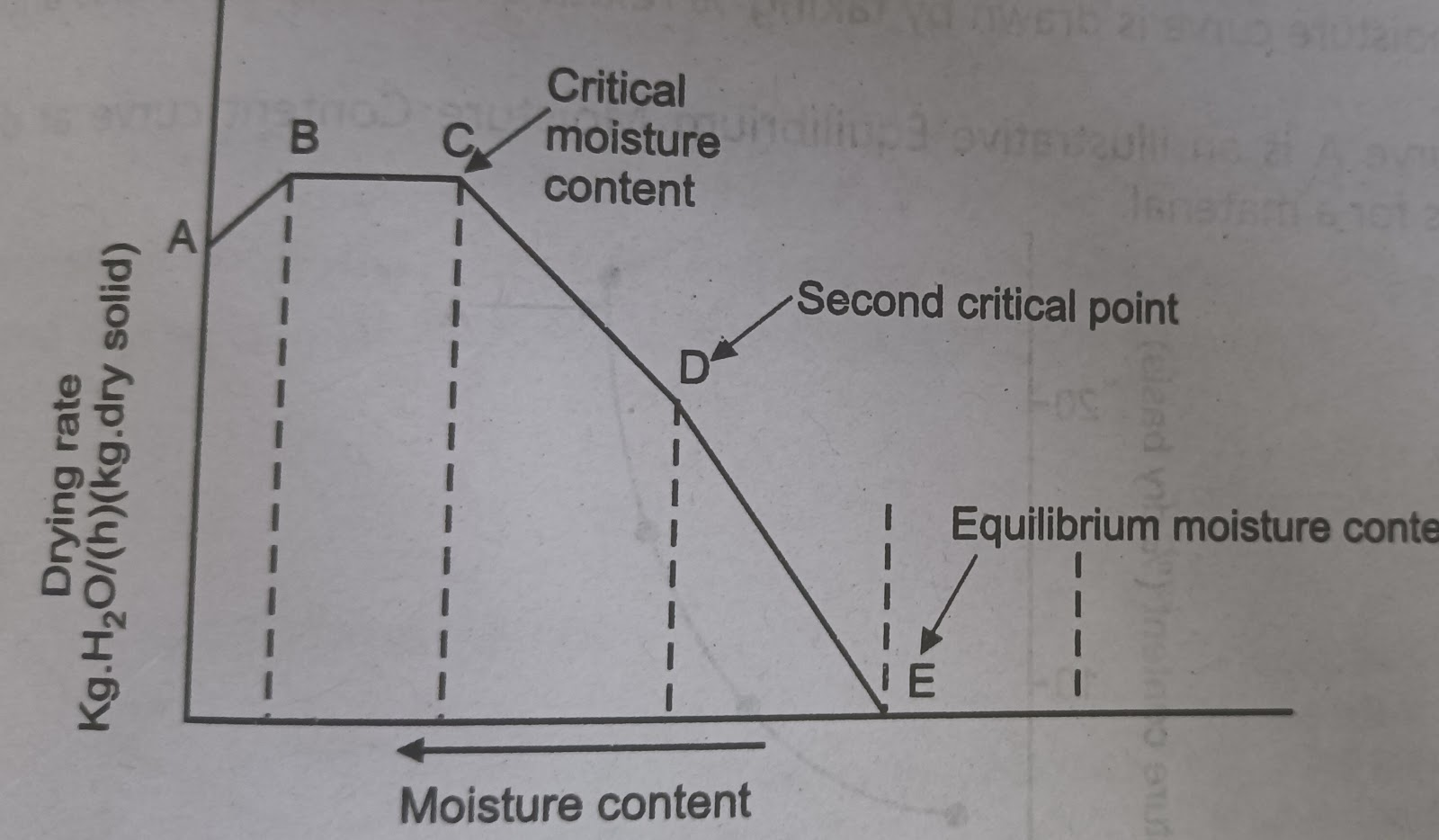### Definition

• Drying is defined as the removal of liquid from a product usually with application of heat.

## Rate of Drying Curve.• Drying process can be divided into three periods

2. Constant drying rate period.

3. First falling drying rate period.

4. Second falling rate period.

1. ### Initial Adjustment Period (A-B):

• Also called the “Heating up” period.

• In this period the substance gets heat and increases in temperature.

• Drying has not yet started.

1. ### Constant drying rate period (B-C):

• During this period the temperature of the solid and the rate of drying remain constant.

• The moisture evaporating from the surface is replaced by water diffusing from the interior of the solid at a rate equal to the rate of evaporation.

• The moisture content at the end of constant rate (point C) is referred to as the critical moisture content (CMC).

• At CMC, dry spots start appearing and drying rate starts falling.

2. ### First falling drying rate period (C-D):

• This period is also called the period of unsaturated surface drying

• During this period the rate of evaporation is insufficient to saturate the air in contact with the surface.

• This is because the surface water is no longer replaced at a rate required to maintain continuous film on the surface.

• Between points C and D, the number and area of dry spots continue to increase, and the rate of drying falls steadily.

• Point D is referred to as the second critical point, at this point, the film of surface water has completely evaporated.

3. ### Second falling rate period (D-E):

• At the end of the first falling rate period, the rate of drying falls even more rapidly.

• The plane of vapourization moves From the surface into the body of the solid.

• The evaporation takes place from within the solid and the vapour reaches the surface by molecular diffusion through the material.

• Point E is referred to as the equilibrium moisture content.

1. ### Equilibrium moisture content (EMC):

• Beyond this point drying is not possible as remaining moisture is bound moisture.

• Drying rate here becomes zero.